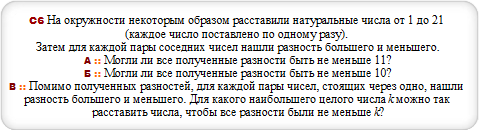# Закажи себе WolframAlpha|Pro! Получи пошаговые решения твоих задач!

## ЕГЭ с Wolfram|Alpha :: 28.04.14 :: Досрочный 1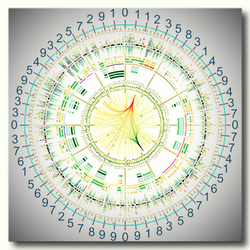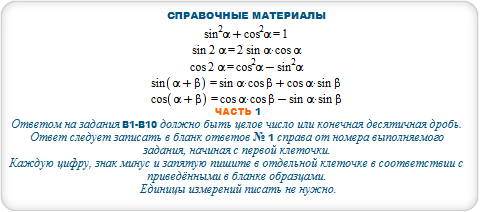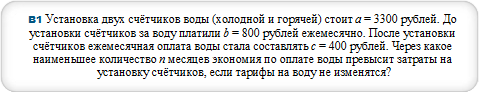#### ceil(a/(b-c)) for a = 3300, b = 800, c=400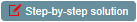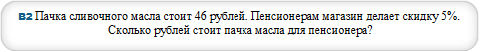#### b=N[a*(1-p/100)] where a=46,p=5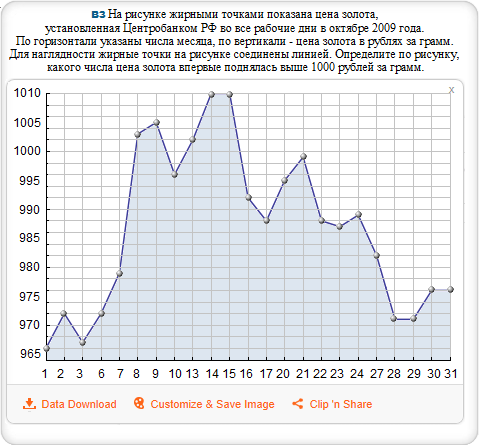#### plot {966,972,967,972,979,1003,1005,996,1002,1010,1010, 992,988,995,999,988,987,989,982,971,971,976,976}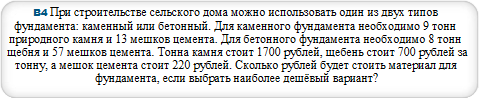#### B=m*v+n*w where m=8,n=57,v=700,w=220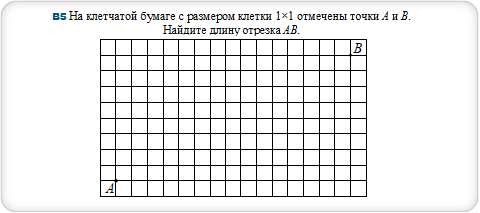#### triangle (1,1),(16,1),(16,9)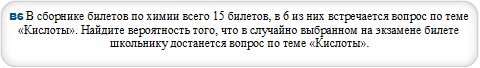#### p=N[m/n] where m=6,n=15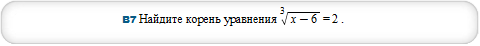#### solve (x-6)^(1/3)=2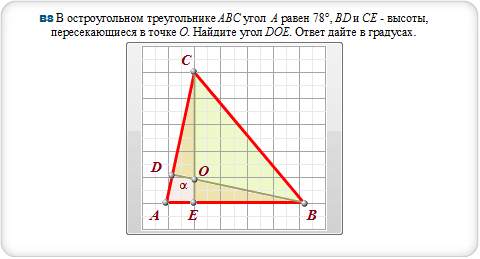#### polygon (0,0),(-2.1,0),(0,10),(8.4,0),(0,0),(0,10),triangle (-2.1,0),(-1.7,2.1),(8.4,0)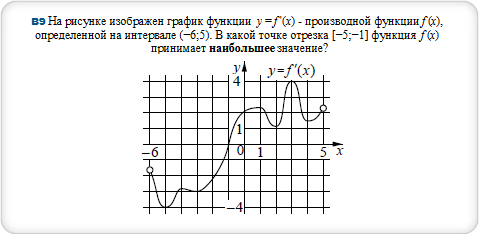#### fit polynomial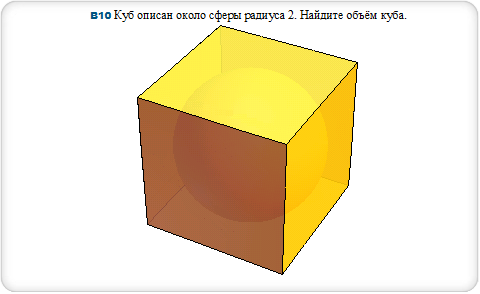#### sphere radius 2, cube edge length 4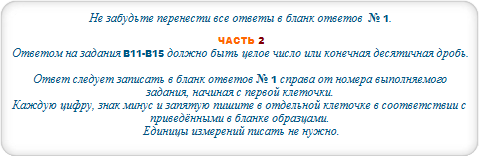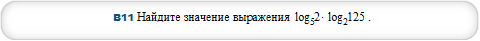#### log(5,2)*log(2,125)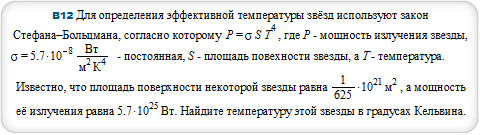#### T = (P/(sigma*S))^(1/4) where P = (57/10)*10^25, sigma = (57/10)*10^(-8), S = (1/625)*10^21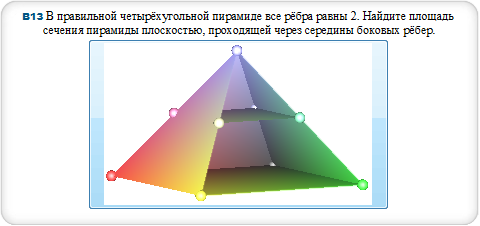#### equilateral square pyramid base 1,height 1/sqrt2 and equilateral square pyramid base 2,height 2/sqrt2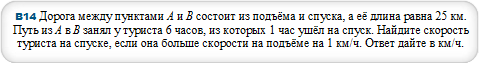#### v=(a*(T-t)+s)/T where s=25,T=6,t=1,a=1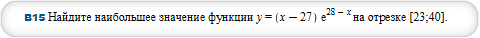#### maximize (x-27)*E^(28-x) over [23,40]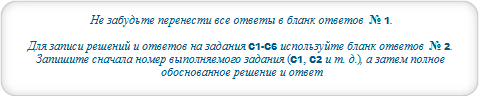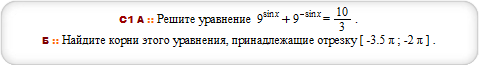#### solve {9^sin(x)+9^(-sin(x)) = 10/3,-7*Pi*(1/2)<=x,x<=-2*Pi}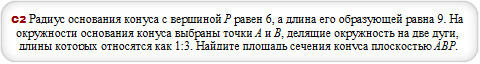#### cone with radius 6, height 3sqrt5 and plane (-6,0,0),(0,-6,0),(0,0,3sqrt5)#### solve {3^x+54/3^x>=29,log(x+3,(x+1)/4)<=0}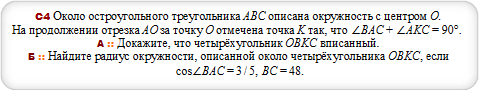#### polygon [7.1, -29.1],[-30, 0],[26, 15],[7.1, -29.1],[46, 0], [26, 15] and circle through [7.1, -29.1],[-30, 0],[26, 15]#### sqrt(x^4+(a-5)^4) = abs(x+a-5)+abs(x-a+5)